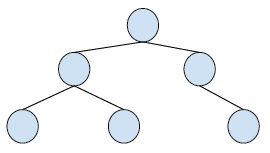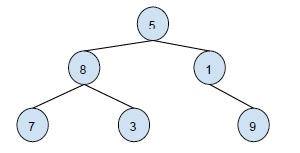# Print Ancestors of a given node in Binary Tree in C++

In this problem, we are given a binary tree and we have to print its ancestor of a node in a binary tree.

Binary Tree is a special tree whose every node has at max two child nodes. So, every node is either a leaf node or has one or two child nodes.

Example,The ancestor of a node in a binary tree is a node that is at the upper level of the given node.

Let’s take an example of ancestor node −Ancestors of a node with value 3 in this binary tree are 8,

For solving this problem, we will traverse from the root node to the target node. Step by step downwards in the binary tree. And in the path print all the nodes that come.

## Example

Live Demo

#include<iostream>
#include<stdio.h>
#include<stdlib.h>
using namespace std;
struct node{
int data;
struct node* left;
struct node* right;
};
bool AncestorsNodes(struct node *root, int target){
if (root == NULL)
return false;
if (root->data == target)
return true;
if ( AncestorsNodes(root->left, target) || AncestorsNodes(root->right, target) ){
cout << root->data << " ";
return true;
}
return false;
}
struct node* insertNode(int data){
struct node* node = (struct node*) malloc(sizeof(struct node));
node->data = data;
node->left = NULL;
node->right = NULL;
return(node);
}
int main(){
struct node *root = insertNode(10);
root->left = insertNode(6);
root->right = insertNode(13);
root->left->left = insertNode(3);
root->left->right = insertNode(8);
root->right->left = insertNode(12);
cout<<"Ancestor Nodes are " ;
AncestorsNodes(root, 8);
getchar();
return 0;
}

## Output

Ancestor Nodes are 6 10